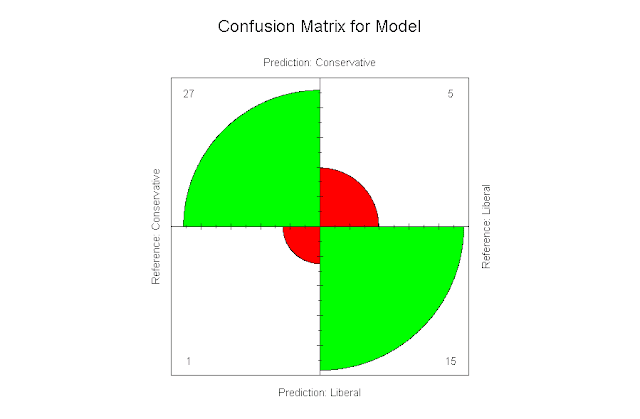### Attractive Confusion Matrices in R Plotted with fourfoldplot

As part of writing analyses using neural networks I thought of displaying a confusion matrix, but went looking for something more. What I found was either ugly and simple, or attractive but complicated. The following code demonstrates using fourfoldplot, with original insight gained from fourfoldplot: A prettier confusion matrix in base R, and a great documentation page, R Documentation's page for fourfoldplot.

The code is below, as is the related graph. Source data can be found here.

`````` ###############################################
# Load and clean data
################################################

Politics.df <- read.csv("BigFiveScoresByState.csv", na.strings = c("", "NA"))
Politics.df <- na.omit(Politics.df)

################################################
# Neural Net with nnet and caret
################################################

# load packages
library(nnet)
library(caret)

# set equation
equation <- as.formula("Liberal ~ Openness + Conscientiousness + Extraversion + Neuroticism + Agreeableness")

# train, predict, combine
Politics.model <- train(equation, Politics.df, method = 'nnet', linout = TRUE, trace = FALSE)
Politics.model.predicted <- predict(Politics.model, Politics.df)
Politics.model.combined <- cbind(Politics.model.predicted, Politics.df)

################################################
# Plot with FourFoldPlot
################################################

# create the confusion matrix
Politics.model.matrix <- confusionMatrix(Politics.model.combined\$predictionBinary, Politics.model.combined\$Liberal)

# set the column and row names
colnames(Politics.model.matrix\$table) <- c("Conservative", "Liberal")
rownames(Politics.model.matrix\$table) <- c("Conservative", "Liberal")

# generate the plot
fourfoldplot(Politics.model.matrix\$table, color = c("Red", "Green"), conf.level = 0, margin = 1, main = "Confusion Matrix for Model")``````